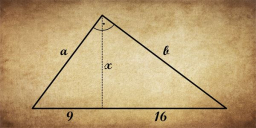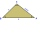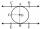# MIT 1869

You know the length of hypotenuse parts 9 and 16, at which the hypotenuse of a right triangle is divided by a perpendicular running from its opposite vertex. The task is to find the lengths of the sides of the triangle and the length of the line x. This assignment was part of the entrance exams to the Massachusetts Institute of Technology MIT in 1869.

c =  25
x =  12
a =  15
b =  20

### Step-by-step explanation:

Try calculation via our triangle calculator.Did you find an error or inaccuracy? Feel free to write us. Thank you!

Showing 1 comment:Peter
Easier solution with almost no calculating. Because all 3 entered triangles are similar, the following holds: x / 9 = 16 / x => x = 12. And because every triangle that has an aspect ratio of 3: 4: 5 is right, it must hold: a = 3 * 5 = 15 ab = 4 * 5 = 20.
PS: Maybe at that time they wanted to know at MIT who was just calculate and who was even thinking.

4 months ago  1 LikeTips to related online calculators
Pythagorean theorem is the base for the right triangle calculator.

#### You need to know the following knowledge to solve this word math problem:

We encourage you to watch this tutorial video on this math problem:

## Related math problems and questions:

• Hypotenuse - RTA triangle has a hypotenuse of 55 and an altitude to the hypotenuse of 33. What is the area of the triangle?
• Speed of Slovakian trainsRudolf decided to take the train from the station 'Ostratice' to 'Horné Ozorovce'. In the train timetables found train Os 5409 : km 0 Chynorany 15:17 5 Ostratice 15:23 15:23 8 Rybany 15:27 15:27 10 Dolné Naštice 15:31 15:31 14 Bánovce nad Bebravou 15:35 1
• Isosceles triangleIn an isosceles triangle ABC with base AB; A [3,4]; B [1,6] and the vertex C lies on the line 5x - 6y - 16 = 0. Calculate the coordinates of vertex C.
• TV diagonalDiagonal TV is 0.56 m long, how big the television sreen is if the aspect ratio is 16:9?
• Right 24Right isosceles triangle has an altitude x drawn from the right angle to the hypotenuse dividing it into 2 unequal segments. The length of one segment is 5 cm. What is the area of the triangle? Thank you.
• Trapezoid RTThe plot has a shape of a rectangular trapezium ABCD, where ABIICD with a right angle at the vertex B. side AB has a length of 36 m. The lengths of the sides AB and BC are in the ratio 12:7. Lengths of the sides AB and CD are a ratio of 3:2. Calculate con
• Z9–I–4 MO 2017Numbers 1, 2, 3, 4, 5, 6, 7, 8 and 9 were prepared for a train journey with three wagons. They wanted to sit out so that three numbers were seated in each carriage and the largest of each of the three was equal to the sum of the remaining two. The conduct
• Widescreen monitorComputer businesses hit by a wave of widescreen monitors and televisions. Calculate the area of ​​the LCD monitor with a diagonal size 20 inches at ratio 4:3 and then 16:9 aspect ratio. Is buying widescreen monitors with the same diagonal more advantageou
• Triangle IRTAn isosceles right triangle ABC with right angle at vertex C has vertex coordinates: A (-1, 2); C (-5, -2) Calculate the length of segment AB.
• Axial section of the coneThe axial section of the cone is an isosceles triangle in which the ratio of cone diameter to cone side is 2: 3. Calculate its volume if you know its area is 314 cm square.
• Right triangle - ratioThe lengths of the legs of the right triangle ABC are in ratio b = 2: 3. The hypotenuse is 10 cm long. Calculate the lengths of the legs of that triangle.
• The trianglesThe triangles KLM and ABC are given, which are similar to each other. Calculate the lengths of the remaining sides of the triangle KLM, if the lengths of the sides are a = 7 b = 5.6 c = 4.9 k = 5
• Free space in the gardenThe grandfather's free space in the garden was in the shape of a rectangular triangle with 5 meters and 12 meters in length. He decided to divide it into two parts and the height of the hypotenuse. For the smaller part creates a rock garden, for the large
• MO - trianglesOn the AB and AC sides of the triangle ABC lies successive points E and F, on segment EF lie point D. The EF and BC lines are parallel and is true this ratio FD:DE = AE:EB = 2:1. The area of ABC triangle is 27 hectares and line segments EF, AD, and DB seg
• Similarity coefficientIn the triangle TMA the length of the sides is t = 5cm, m = 3.5cm, a = 6.2cm. Another similar triangle has side lengths of 6.65 cm, 11.78 cm, 9.5 cm. Determine the similarity coefficient of these triangles and assign similar sides to each other.
• Rectangular trianglesThe lengths of corresponding sides of two rectangular triangles are in the ratio 2:5. At what ratio are medians relevant to hypotenuse these right triangles? At what ratio are the contents of these triangles? Smaller rectangular triangle has legs 6 and 8
• Tangent 3In a circle with centre O radius is 4√5 cm. EC is the tangent to the circle at point D. Segment AB IS THE DIAMETER of given circle. POINT A is joined with POINT E and POINT B is joined with POINT C. Find DC if BC IS 8cm.